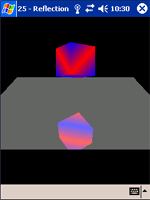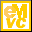Home Latest News Archive Tutorials C++ 2 3 4 Win32 Java OpenGL GLUT OpenGL ES 2 3 4 DirectX VB.NET HTML Photoshop 3dsmax Forums Projects Modelling Links About Us Search Recent Tutorials C / C++ Win32 OpenGL OpenGL ES GLUT## Reflections

Tutorials > OpenGL ES > Reflections

### IntroductionIn the real world, reflections are always evident. This tutorial will explain how to create reflections using the stencil buffer.

### How does the Stencil Buffer Work?

The stencil buffer buffer is used to stop primitives from being rendered in certain parts of the scene. We will be setting up the stencil buffer so that a pixel with a stencil value of 0 will not be displayed and a pixel with a stencil value of 1 will be displayed.

Without the stencil buffer, we would still be able to show the reflected block, although the block would be entirely rendered instead of only being rendered in the floor.

This tutorial is built upon tutorial 14 on Backface Culling.

Contents of main.cpp :

We create an x and y rotation variable to rotate the box as per normal. We also create 2 more variables which will be used to make the cube move up and down while it is rotating. This helps to show off the reflection effect more.

```float xrot = 0.0f;
float yrot = 0.0f;
float yval = 1.0f;
bool yup = true;
```

We will be displaying a cube that is reflected in a floor. This floor must be placed on the y = 0 axis as we are going to simply flip the cubes y position to render the reflected block.

```GLfloat floorVertices[] = {
-3.0f, 0.0f, 3.0f,
3.0f, 0.0f, 3.0f,
-3.0f, 0.0f,-3.0f,
3.0f, 0.0f,-3.0f
};
```

Our init function starts off as per normal.

```void init()
{
glEnable(GL_DEPTH_TEST);
glDepthFunc(GL_LEQUAL);

glClearColor(0.0f, 0.0f, 0.0f, 0.0f);
glClearDepthf(1.0f);
```

As with the color and depth buffers, we also need to specify what the stencil buffer should be cleared with. This is achieved by using the glClearStencil function. This function takes one parameter, being the value that we want to use to clear the stencil buffer with. We want to start off with not allowing any primitives to be drawn anywhere. The clearing value of 0 is therefore passed.

```	glClearStencil(0);

glEnableClientState(GL_VERTEX_ARRAY);
glColorPointer(4, GL_FLOAT, 0, boxColors);

glEnable(GL_CULL_FACE);
}
```

To create the reflection illusion, we need to draw the cube twice. It is therefore easier to create a function that will draw a cube.

```void drawCube()
{
```

We push the current matrix on the stack so that it can be reset when we are finished. We also enable the color array.

```	glPushMatrix();

glEnableClientState(GL_COLOR_ARRAY);
```

Here we use the yval variable to place the cube at different heights.

```	glTranslatef(0.0f, yval, 0.0f);

glRotatef(xrot, 1.0f, 0.0f, 0.0f);
glRotatef(yrot, 0.0f, 1.0f, 0.0f);

glVertexPointer(3, GL_FLOAT, 0, box);

glDrawArrays(GL_TRIANGLE_STRIP, 0, 4);
glDrawArrays(GL_TRIANGLE_STRIP, 4, 4);

glDrawArrays(GL_TRIANGLE_STRIP, 8, 4);
glDrawArrays(GL_TRIANGLE_STRIP, 12, 4);

glDrawArrays(GL_TRIANGLE_STRIP, 16, 4);
glDrawArrays(GL_TRIANGLE_STRIP, 20, 4);

glDisableClientState(GL_COLOR_ARRAY);

glPopMatrix();
}
```

Our display function sees the most change.

```void display()
{
```

As with all the buffers, the first step to take is to clear the stencil buffer. This is done by passing GL_STENCIL_BUFFER_BIT to the the glClear function.

```	glClear(GL_COLOR_BUFFER_BIT | GL_DEPTH_BUFFER_BIT
| GL_STENCIL_BUFFER_BIT);
```

We want to place the camera above and to the right of the floor, while looking down upon it.

```	gluLookAtf(
0.0f, 3.0f, 7.0f,
0.0f, 0.0f, 0.0f,
0.0f, 1.0f, 0.0f);
```

When writing to the stencil buffer, we do not want to display anything to the screen. We therefore need to make a call to the glColorMask function. This function takes 4 parameters. Each paramater can take either GL_TRUE or GL_FALSE. Each parameter specifies whether the red, green, blue and alpha color values can be written to.

```	glColorMask(GL_FALSE, GL_FALSE, GL_FALSE, GL_FALSE);
```

We also do not want to write to our depth buffer, so we make a call to glDepthMask.

```	glDepthMask(GL_FALSE);
```

The stencil buffer also has a function like the ones used above. This is the glStencilMask function and accepts the same parameter as the glDepthMask function.

Like with depth testing, we need to enable stencil testing. This is done by passing GL_STENCIL_TEST onto the glEnable function.

```	glEnable(GL_STENCIL_TEST);
```

One of the main stencil functions is the glStencilOp function. This function takes 3 parameters which can be the values GL_KEEP, GL_ZERO, GL_REPLACE, GL_INCR, GL_DECR or GL_INVERT. The default value is GL_KEEP. Each parameter is explained below :

GLenum fail - This specifies the action to take when the stencil test fails.

GLenum zfail - This specifies the action to take when the stencil test passes but the depth test fails.

GLenum zpass - This specifies the action to take when both the stencil and depth tests pass.

At the moment, all our buffers are cleared. We want to place entries in the stencil buffer wherever we draw the next set of primitives. We have disabled writing to the color and depth buffers, so the only one being written to is the stencil buffer.

We therefore pass GL_REPLACE as all 3 parameters to the glStencilOp function. This makes sure that we always write to the stencil buffer.

```	glStencilOp(GL_REPLACE, GL_REPLACE, GL_REPLACE);
```

Our next main stencil function is the glStencilFunc function. This specifies how the stencil test should be made. The parameters are explained below :

GLenum func - This specifies how the stencil comparison should be made. This value can be any of the values that is normally passed onto the glDepthFunc function eg. GL_LESS, GL_LEQUAL, etc.
We want the stencil test to always pass at this point in time, so we pass GL_ALWAYS as our first parameter.

GLint ref - This is the value that is tested against. We want this value to be 1.

GLuint mask - This specifies the stencil mask. This value is ANDed to the reference value given above when placing the value in the stencil buffer. We want the value that must be placed in the stencil buffer to be 1. We therefore use 1 for this parameter as 1 & 1 is 1.

```	glStencilFunc(GL_ALWAYS, 1, 1);
```

We now "draw" the floor. The floor will not actually be displayed, but the stencil buffer will contain a 1 for every pixel of the floor.

```	// Floor
glVertexPointer(3, GL_FLOAT, 0, floorVertices);
glColor4f(1.0f, 1.0f, 1.0f, 1.0f);
glDrawArrays(GL_TRIANGLE_STRIP, 0, 4);
```

Now that we have initialized our stencil buffer, we can actually draw something to the screen.

It is therefore necessary to enable writing to both the color and depth buffers once again.

```	glColorMask(GL_TRUE, GL_TRUE, GL_TRUE, GL_TRUE);
```

The reflection illusion is essentially created by flipping the cube and then drawing it. When our cube is near the floor, the entire cube is shown in the reflection. When the cube is in the sky, the cubes reflection needs to appear only slightly in the floor. We now want to only draw the mirrored cube in the floor.

The first step is to pass GL_EQUAL onto the glStencilFunc function along with a reference and a mask of 1. This will cause the stencil test to only pass if the value in the stencil buffer is equal to 1.

```	glStencilFunc(GL_EQUAL, 1, 1);
```

We do not want the values in the stencil buffer to be modified, so we pass GL_KEEP for all parameters of the glStencilOp function.

```	glStencilOp(GL_KEEP, GL_KEEP, GL_KEEP);
```

It is now time to draw our mirrored cube.

```	// Cube
glPushMatrix();
{
```

An easy way to flip the cube is to make a call to glScalef with all parameters 1 except that the y value must be given as -1.

```		glScalef(1.0f, -1.0f, 1.0f);
```

Because you are flipping the cube, the front faces are reversed. This requires the need to not cull back faces, but rather cull front faces. This is achieved by passing GL_FRONT onto the glCullFace function as we have seen before.

```		glCullFace(GL_FRONT);

drawCube();
```

Once we have rendered the reflected cube, we need to reset culling for backfacing polygons.

```		glCullFace(GL_BACK);
}
glPopMatrix();
```

Our reflection has been done so we do not need the stencil buffer anymore. We can therefore disable stencil testing.

```	glDisable(GL_STENCIL_TEST);
```

We want to display a floor that is transparent, allowing us to see the mirrored cube below. As we have seen in the past, this can be achieved by enabling blending with our standard transparent blend function.

```	glEnable(GL_BLEND);
glBlendFunc(GL_SRC_ALPHA, GL_ONE_MINUS_SRC_ALPHA);
```

The floor is rendered with an alpha value of 0.4.

```	// Floor
glVertexPointer(3, GL_FLOAT, 0, floorVertices);
glColor4f(1.0f, 1.0f, 1.0f, 0.4f);
glDrawArrays(GL_TRIANGLE_STRIP, 0, 4);
```

The last step is to disable blending and draw our original cube.

```	glDisable(GL_BLEND);

drawCube();

glFlush();
glutSwapBuffers();
}
```

Our animation code needs to appear in the idle function. The function starts off with the standard increase of rotation variables.

```void idle()
{
xrot += 2.0f;
yrot += 3.0f;
```

The y position of the cube is changed depending on whether it is moving up or down.

```	yval += yup ? 0.02f : -0.02f;
```

The direction also needs to be changed if the cube moves below 1 or above 2.

```	if (yval > 2.0f)
yup = false;
if (yval < 1.0f)
yup = true;

glutPostRedisplay();
}
```

Well done. This was quite a long tutorial. Hopefully you will now understand how the stencil buffer works. The stencil buffer can also be used for other effects such as shadows. We will cover some other effects in future tutorials.

Please let me know of any comments you may have : Contact Me

 GLUT|ES Source Files :UG Source Files :Last Updated : 20 November 2005